# Thermodynamics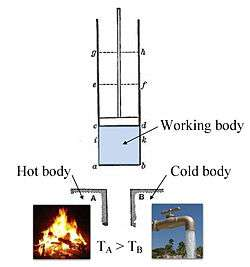Annotated color version of the original 1824 Carnot heat engine showing the hot body (boiler), working body (system, steam), and cold body (water), the letters labeled according to the stopping points in Carnot cycle.

Thermodynamics is a branch of science concerned with heat and temperature and their relation to energy and work. The behavior of these quantities is governed by the four laws of thermodynamics, irrespective of the composition or specific properties of the material or system in question. The laws of thermodynamics are explained in terms of microscopic constituents by statistical mechanics. Thermodynamics applies to a wide variety of topics in science and engineering, especially physical chemistry, chemical engineering and mechanical engineering.

Historically, thermodynamics developed out of a desire to increase the efficiency of early steam engines, particularly through the work of French physicist Nicolas Léonard Sadi Carnot (1824) who believed that engine efficiency was the key that could help France win the Napoleonic Wars. Scottish physicist Lord Kelvin was the first to formulate a concise definition of thermodynamics in 1854:

Thermo-dynamics is the subject of the relation of heat to forces acting between contiguous parts of bodies, and the relation of heat to electrical agency.

The initial application of thermodynamics to mechanical heat engines was extended early on to the study of chemical systems. Chemical thermodynamics studies the nature of the role of entropy in the process of chemical reactions and provided the bulk of expansion and knowledge of the field. Other formulations of thermodynamics emerged in the following decades. Statistical thermodynamics, or statistical mechanics, concerned itself with statistical predictions of the collective motion of particles from their microscopic behavior. In 1909, Constantin Carathéodory presented a purely mathematical approach to the field in his axiomatic formulation of thermodynamics, a description often referred to as geometrical thermodynamics.

## Introduction

The starting point for most considerations of thermodynamic systems are the laws of thermodynamics, four principles that form an axiomatic basis. The first law specifies that energy can be exchanged between physical systems as heat and work. The second law defines the existence of the quantity entropy, a quantification of the state of order of a system that expresses the notion of useful work that can be performed.

In thermodynamics, interactions between large ensembles of objects are studied and categorized. Central to this are the concepts of system and surroundings. A system is composed of particles, whose average motions define its properties, which in turn are related to one another through equations of state. Properties can be combined to express internal energy and thermodynamic potentials, which are useful for determining conditions for equilibrium and spontaneous processes.

With these tools, thermodynamics can be used to describe how systems respond to changes in their environment. This can be applied to a wide variety of topics in science and engineering, such as engines, phase transitions, chemical reactions, transport phenomena, and even black holes. The results of thermodynamics are essential for other fields of physics and for chemistry, chemical engineering, aerospace engineering, mechanical engineering, cell biology, biomedical engineering, materials science, and economics, to name a few.

This article is focused mainly on classical thermodynamics which primarily studies systems in thermodynamic equilibrium. Non-equilibrium thermodynamics is often treated as an extension of the classical treatment, but statistical mechanics has brought many advances of the field.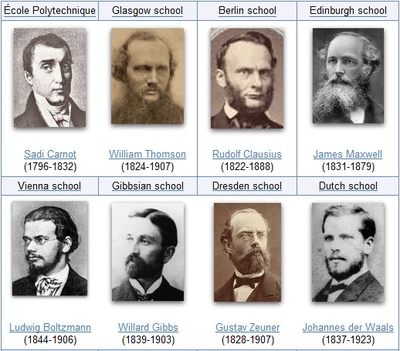The thermodynamicists representative of the original eight founding schools of thermodynamics. The schools with the most-lasting effect in founding the modern versions of thermodynamics are the Berlin school, particularly as established in Rudolf Clausius’s 1865 textbook The Mechanical Theory of Heat, the Vienna school, with the statistical mechanics of Ludwig Boltzmann, and the Gibbsian school at Yale University, American engineer Willard Gibbs' 1876 On the Equilibrium of Heterogeneous Substances launching chemical thermodynamics.

## History

The history of thermodynamics as a scientific discipline generally begins with Otto von Guericke who, in 1650, built and designed the world's first vacuum pump and demonstrated a vacuum using his Magdeburg hemispheres. Guericke was driven to make a vacuum in order to disprove Aristotle's long-held supposition that 'nature abhors a vacuum'. Shortly after Guericke, the English physicist and chemist Robert Boyle had learned of Guericke's designs and, in 1656, in coordination with English scientist Robert Hooke, built an air pump. Using this pump, Boyle and Hooke noticed a correlation between pressure, temperature, and volume. In time, Boyle's Law was formulated, which states that pressure and volume are inversely proportional. Then, in 1679, based on these concepts, an associate of Boyle's named Denis Papin built a steam digester, which was a closed vessel with a tightly fitting lid that confined steam until a high pressure was generated.

Later designs implemented a steam release valve that kept the machine from exploding. By watching the valve rhythmically move up and down, Papin conceived of the idea of a piston and a cylinder engine. He did not, however, follow through with his design. Nevertheless, in 1697, based on Papin's designs, engineer Thomas Savery built the first engine, followed by Thomas Newcomen in 1712. Although these early engines were crude and inefficient, they attracted the attention of the leading scientists of the time.

The fundamental concepts of heat capacity and latent heat, which were necessary for the development of thermodynamics, were developed by Professor Joseph Black at the University of Glasgow, where James Watt was employed as an instrument maker. Black and Watt performed experiments together, but it was Watt who conceived the idea of the external condenser which resulted in a large increase in steam engine efficiency. Drawing on all the previous work led Sadi Carnot, the "father of thermodynamics", to publish Reflections on the Motive Power of Fire (1824), a discourse on heat, power, energy and engine efficiency. The paper outlined the basic energetic relations between the Carnot engine, the Carnot cycle, and motive power. It marked the start of thermodynamics as a modern science.

The first thermodynamic textbook was written in 1859 by William Rankine, originally trained as a physicist and a civil and mechanical engineering professor at the University of Glasgow. The first and second laws of thermodynamics emerged simultaneously in the 1850s, primarily out of the works of William Rankine, Rudolf Clausius, and William Thomson (Lord Kelvin).

The foundations of statistical thermodynamics were set out by physicists such as James Clerk Maxwell, Ludwig Boltzmann, Max Planck, Rudolf Clausius and J. Willard Gibbs.

During the years 1873-76 the American mathematical physicist Josiah Willard Gibbs published a series of three papers, the most famous being On the Equilibrium of Heterogeneous Substances, in which he showed how thermodynamic processes, including chemical reactions, could be graphically analyzed, by studying the energy, entropy, volume, temperature and pressure of the thermodynamic system in such a manner, one can determine if a process would occur spontaneously. Also Pierre Duhem in the 19th century wrote about chemical thermodynamics. During the early 20th century, chemists such as Gilbert N. Lewis, Merle Randall, and E. A. Guggenheim applied the mathematical methods of Gibbs to the analysis of chemical processes.

## Etymology

The etymology of thermodynamics has an intricate history. It was first spelled in a hyphenated form as an adjective (thermo-dynamic) and from 1854 to 1868 as the noun thermo-dynamics to represent the science of generalized heat engines.

American biophysicist Donald Haynie claims that thermodynamics was coined in 1840 from the Greek root θέρμη therme, meaning heat and δύναμις dynamis, meaning power. However, this etymology has been cited as unlikely.

Pierre Perrot claims that the term thermodynamics was coined by James Joule in 1858 to designate the science of relations between heat and power, however, Joule never used that term, but used instead the term perfect thermo-dynamic engine in reference to Thomson’s 1849 phraseology.

By 1858, thermo-dynamics, as a functional term, was used in William Thomson's paper An Account of Carnot's Theory of the Motive Power of Heat.

## Branches of description

The study of thermodynamical systems has developed into several related branches, each using a different fundamental model as a theoretical or experimental basis, or applying the principles to varying types of systems.

### Classical thermodynamics

Classical thermodynamics is the description of the states of thermodynamical systems at near-equilibrium, using macroscopic, empirical properties directly measurable in the laboratory. It is used to model exchanges of energy, work and heat based on the laws of thermodynamics. The qualifier classical reflects the fact that it represents the descriptive level in terms of macroscopic empirical parameters that can be measured in the laboratory, that was the first level of understanding in the 19th century. A microscopic interpretation of these concepts was provided by the development of statistical mechanics.

### Statistical mechanics

Statistical mechanics, also called statistical thermodynamics, emerged with the development of atomic and molecular theories in the late 19th century and early 20th century, supplementing thermodynamics with an interpretation of the microscopic interactions between individual particles or quantum-mechanical states. This field relates the microscopic properties of individual atoms and molecules to the macroscopic, bulk properties of materials that can be observed on the human scale, thereby explaining thermodynamics as a natural result of statistics, classical mechanics, and quantum theory at the microscopic level.

### Chemical thermodynamics

Chemical thermodynamics is the study of the interrelation of energy with chemical reactions or with a physical change of state within the confines of the laws of thermodynamics.

### Treatment of equilibrium

Equilibrium thermodynamics is the systematic study of transformations of matter and energy in systems as they approach equilibrium. The word equilibrium implies a state of balance. In an equilibrium state there are no unbalanced potentials, or driving forces, within the system. A central aim in equilibrium thermodynamics is: given a system in a well-defined initial state, subject to accurately specified constraints, to calculate what the state of the system will be once it has reached equilibrium.

Non-equilibrium thermodynamics is a branch of thermodynamics that deals with systems that are not in thermodynamic equilibrium. Most systems found in nature are not in thermodynamic equilibrium because they are not in stationary states, and are continuously and discontinuously subject to flux of matter and energy to and from other systems. The thermodynamic study of non-equilibrium systems requires more general concepts than are dealt with by equilibrium thermodynamics. Many natural systems still today remain beyond the scope of currently known macroscopic thermodynamic methods.

## Laws of thermodynamics

Thermodynamics is principally based on a set of four laws which are universally valid when applied to systems that fall within the constraints implied by each. In the various theoretical descriptions of thermodynamics these laws may be expressed in seemingly differing forms, but the most prominent formulations are the following:

• Zeroth law of thermodynamics: If two systems are each in thermal equilibrium with a third, they are also in thermal equilibrium with each other.

This statement implies that thermal equilibrium is an equivalence relation on the set of thermodynamic systems under consideration. Systems are said to be in equilibrium if the small, random exchanges between them (e.g. Brownian motion) do not lead to a net change in energy. This law is tacitly assumed in every measurement of temperature. Thus, if one seeks to decide if two bodies are at the same temperature, it is not necessary to bring them into contact and measure any changes of their observable properties in time. The law provides an empirical definition of temperature and justification for the construction of practical thermometers.

The zeroth law was not initially recognized as a law, as its basis in thermodynamical equilibrium was implied in the other laws. The first, second, and third laws had been explicitly stated prior and found common acceptance in the physics community. Once the importance of the zeroth law for the definition of temperature was realized, it was impracticable to renumber the other laws, hence it was numbered the zeroth law.

The first law of thermodynamics is an expression of the principle of conservation of energy. It states that energy can be transformed (changed from one form to another), but cannot be created or destroyed.

The first law is usually formulated by saying that the change in the internal energy of a closed thermodynamic system is equal to the difference between the heat supplied to the system and the amount of work done by the system on its surroundings. It is important to note that internal energy is a state of the system (see Thermodynamic state) whereas heat and work modify the state of the system. In other words, a specific internal energy of a system may be achieved by any combination of heat and work; the manner by which a system achieves a specific internal energy is path independent.

The second law of thermodynamics is an expression of the universal principle of decay observable in nature. The second law is an observation of the fact that over time, differences in temperature, pressure, and chemical potential tend to even out in a physical system that is isolated from the outside world. Entropy is a measure of how much this process has progressed. The entropy of an isolated system which is not in equilibrium will tend to increase over time, approaching a maximum value at equilibrium.

In classical thermodynamics, the second law is a basic postulate applicable to any system involving heat energy transfer; in statistical thermodynamics, the second law is a consequence of the assumed randomness of molecular chaos. There are many versions of the second law, but they all have the same effect, which is to explain the phenomenon of irreversibility in nature.

• Third law of thermodynamics: As a system approaches absolute zero, all processes cease and the entropy of the system approaches a minimum value.

The third law of thermodynamics is a statistical law of nature regarding entropy and the impossibility of reaching absolute zero of temperature. This law provides an absolute reference point for the determination of entropy. The entropy determined relative to this point is the absolute entropy. Alternate definitions are, "the entropy of all systems and of all states of a system is smallest at absolute zero," or equivalently "it is impossible to reach the absolute zero of temperature by any finite number of processes".

Absolute zero, at which all activity would stop if it were possible to happen, is −273.15 °C (degrees Celsius), or −459.67 °F (degrees Fahrenheit) or 0 K (kelvin).

## System models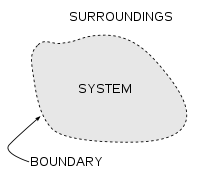A diagram of a generic thermodynamic system

An important concept in thermodynamics is the thermodynamic system, a precisely defined region of the universe under study. Everything in the universe except the system is known as the surroundings. A system is separated from the remainder of the universe by a boundary which may be notional or not, but which by convention delimits a finite volume. Exchanges of work, heat, or matter between the system and the surroundings take place across this boundary.

In practice, the boundary is simply an imaginary dotted line drawn around a volume when there is going to be a change in the internal energy of that volume. Anything that passes across the boundary that affects a change in the internal energy needs to be accounted for in the energy balance equation. The volume can be the region surrounding a single atom resonating energy, such as Max Planck defined in 1900; it can be a body of steam or air in a steam engine, such as Sadi Carnot defined in 1824; it can be the body of a tropical cyclone, such as Kerry Emanuel theorized in 1986 in the field of atmospheric thermodynamics; it could also be just one nuclide (i.e. a system of quarks) as hypothesized in quantum thermodynamics.

Boundaries are of four types: fixed, moveable, real, and imaginary. For example, in an engine, a fixed boundary means the piston is locked at its position; as such, a constant volume process occurs. In that same engine, a moveable boundary allows the piston to move in and out. For closed systems, boundaries are real while for open system boundaries are often imaginary.

Generally, thermodynamics distinguishes three classes of systems, defined in terms of what is allowed to cross their boundaries:

Interactions of thermodynamic systems
Type of system Mass flow Work Heat
Open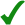Closed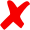NThermally isolatedNN
Mechanically isolatedNNIsolatedNNN

As time passes in an isolated system, internal differences in the system tend to even out and pressures and temperatures tend to equalize, as do density differences. A system in which all equalizing processes have gone to completion is considered to be in a state of thermodynamic equilibrium.

In thermodynamic equilibrium, a system's properties are, by definition, unchanging in time. Systems in equilibrium are much simpler and easier to understand than systems which are not in equilibrium. Often, when analysing a thermodynamic process, it can be assumed that each intermediate state in the process is at equilibrium. This will also considerably simplify the situation. Thermodynamic processes which develop so slowly as to allow each intermediate step to be an equilibrium state are said to be reversible processes.

## States and processes

When a system is at equilibrium under a given set of conditions, it is said to be in a definite thermodynamic state. The state of the system can be described by a number of state quantities that do not depend on the process by which the system arrived at its state. They are called intensive variables or extensive variables according to how they change when the size of the system changes. The properties of the system can be described by an equation of state which specifies the relationship between these variables. State may be thought of as the instantaneous quantitative description of a system with a set number of variables held constant.

A thermodynamic process may be defined as the energetic evolution of a thermodynamic system proceeding from an initial state to a final state. It can be described by process quantities. Typically, each thermodynamic process is distinguished from other processes in energetic character according to what parameters, such as temperature, pressure, or volume, etc., are held fixed. Furthermore, it is useful to group these processes into pairs, in which each variable held constant is one member of a conjugate pair.

Several commonly studied thermodynamic processes are:

## Instrumentation

There are two types of thermodynamic instruments, the meter and the reservoir. A thermodynamic meter is any device which measures any parameter of a thermodynamic system. In some cases, the thermodynamic parameter is actually defined in terms of an idealized measuring instrument. For example, the zeroth law states that if two bodies are in thermal equilibrium with a third body, they are also in thermal equilibrium with each other. This principle, as noted by James Maxwell in 1872, asserts that it is possible to measure temperature. An idealized thermometer is a sample of an ideal gas at constant pressure. From the ideal gas law pV=nRT, the volume of such a sample can be used as an indicator of temperature; in this manner it defines temperature. Although pressure is defined mechanically, a pressure-measuring device, called a barometer may also be constructed from a sample of an ideal gas held at a constant temperature. A calorimeter is a device which is used to measure and define the internal energy of a system.

A thermodynamic reservoir is a system which is so large that it does not appreciably alter its state parameters when brought into contact with the test system. It is used to impose a particular value of a state parameter upon the system. For example, a pressure reservoir is a system at a particular pressure, which imposes that pressure upon any test system that it is mechanically connected to. The Earth's atmosphere is often used as a pressure reservoir.

## Conjugate variables

Main article: Conjugate variables

The central concept of thermodynamics is that of energy, the ability to do work. By the First Law, the total energy of a system and its surroundings is conserved. Energy may be transferred into a system by heating, compression, or addition of matter, and extracted from a system by cooling, expansion, or extraction of matter. In mechanics, for example, energy transfer equals the product of the force applied to a body and the resulting displacement.

Conjugate variables are pairs of thermodynamic concepts, with the first being akin to a "force" applied to some thermodynamic system, the second being akin to the resulting "displacement," and the product of the two equalling the amount of energy transferred. The common conjugate variables are:

## Potentials

Thermodynamic potentials are different quantitative measures of the stored energy in a system. Potentials are used to measure energy changes in systems as they evolve from an initial state to a final state. The potential used depends on the constraints of the system, such as constant temperature or pressure. For example, the Helmholtz and Gibbs energies are the energies available in a system to do useful work when the temperature and volume or the pressure and temperature are fixed, respectively.

The five most well known potentials are:

Name Symbol Formula Natural variables
Internal energyHelmholtz free energyEnthalpyGibbs free energyLandau Potential (Grand potential),whereis the temperature,the entropy,the pressure,the volume,the chemical potential,the number of particles in the system, andis the count of particles types in the system.

Thermodynamic potentials can be derived from the energy balance equation applied to a thermodynamic system. Other thermodynamic potentials can also be obtained through Legendre transformation.

## References

1. Clausius, Rudolf (1850). On the Motive Power of Heat, and on the Laws which can be deduced from it for the Theory of Heat. Poggendorff's Annalen der Physik, LXXIX (Dover Reprint). ISBN 0-486-59065-8.
2. William Thomson, LL.D. D.C.L., F.R.S. (1882). Mathematical and Physical Papers. 1. London, Cambridge: C.J. Clay, M.A. & Son, Cambridge University Press. p. 232.
3. Gibbs, Willard, J. (1876). Transactions of the Connecticut Academy, III, pp. 108-248, Oct. 1875-May 1876, and pp. 343-524, May 1877-July 1878.
4. Duhem, P.M.M. (1886). Le Potential Thermodynamique et ses Applications, Hermann, Paris.
5. Lewis, Gilbert N.; Randall, Merle (1923). Thermodynamics and the Free Energy of Chemical Substances. McGraw-Hill Book Co. Inc.
6. Guggenheim, E.A. (1933). Modern Thermodynamics by the Methods of J.W. Gibbs, Methuen, London.
7. Guggenheim, E.A. (1949/1967). Thermodynamics. An Advanced Treatment for Chemists and Physicists, 1st edition 1949, 5th edition 1967, North-Holland, Amsterdam.
8. Ilya Prigogine, I. & Defay, R., translated by D.H. Everett (1954). Chemical Thermodynamics. Longmans, Green & Co., London. Includes classical non-equilibrium thermodynamics.
9. Enrico Fermi (1956). Thermodynamics. Courier Dover Publications. pp. (ix). ISBN 048660361X. OCLC 230763036.
10. Perrot, Pierre (1998). A to Z of Thermodynamics. Oxford University Press. ISBN 0-19-856552-6. OCLC 123283342.
11. Clark, John, O.E. (2004). The Essential Dictionary of Science. Barnes & Noble Books. ISBN 0-7607-4616-8. OCLC 58732844.
12. Van Ness, H.C. (1983) . Understanding Thermodynamics. Dover Publications, Inc. ISBN 9780486632773. OCLC 8846081.
13. Dugdale, J.S. (1998). Entropy and its Physical Meaning. Taylor and Francis. ISBN 0-7484-0569-0. OCLC 36457809.
14. Smith, J.M.; Van Ness, H.C.; Abbott, M.M. (2005). Introduction to Chemical Engineering Thermodynamics. McGraw Hill. ISBN 0-07-310445-0. OCLC 56491111.
15. Haynie, Donald, T. (2001). Biological Thermodynamics. Cambridge University Press. ISBN 0-521-79549-4. OCLC 43993556.
16. Schools of thermodynamics - EoHT.info.
17. Partington, J.R. (1989). A Short History of Chemistry. Dover. OCLC 19353301.
18. The Newcomen engine was improved from 1711 until Watt's work, making the efficiency comparison subject to qualification, but the increase from the 1865 version was on the order of 100%.
19. Cengel, Yunus A.; Boles, Michael A. (2005). Thermodynamics - an Engineering Approach. McGraw-Hill. ISBN 0-07-310768-9.
20. Gibbs, Willard (1993). The Scientific Papers of J. Willard Gibbs, Volume One: Thermodynamics. Ox Bow Press. ISBN 0-918024-77-3. OCLC 27974820.
21. "Thermodynamics (etymology)". EoHT.info.
22. Donald T. Haynie (2008). Biological Thermodynamics (2 ed.). Cambridge University Press. p. 26.
23. Kelvin, William T. (1849) "An Account of Carnot's Theory of the Motive Power of Heat - with Numerical Results Deduced from Regnault's Experiments on Steam." Transactions of the Edinburg Royal Society, XVI. January 2.Scanned Copy
24. Moran, Michael J. and Howard N. Shapiro, 2008. Fundamentals of Engineering Thermodynamics. 6th ed. Wiley and Sons: 16.
25. "Energy Rules! Energy Conversion and the Laws of Thermodynamics - More About the First and Second Laws". Uwsp.edu. Retrieved 2010-09-12.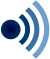Wikiquote has quotations related to: Thermodynamics